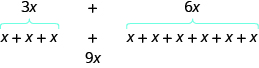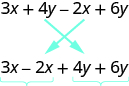## Simplifying Algebraic Expressions

### Learning Outcomes

• Identify the variables and constants in a term
• Identify the coefficient of a variable term
• Identify and combine like terms in an expression

## Identify Terms, Coefficients, and Like Terms

Algebraic expressions are made up of terms. A term is a constant or the product of a constant and one or more variables. Some examples of terms are $7,y,5{x}^{2},9a,\text{and }13xy$.

The constant that multiplies the variable(s) in a term is called the coefficient. We can think of the coefficient as the number in front of the variable. The coefficient of the term $3x$ is $3$. When we write $x$, the coefficient is $1$, since $x=1\cdot x$. The table below gives the coefficients for each of the terms in the left column.

Term Coefficient
$7$ $7$
$9a$ $9$
$y$ $1$
$5{x}^{2}$ $5$

An algebraic expression may consist of one or more terms added or subtracted. In this chapter, we will only work with terms that are added together. The table below gives some examples of algebraic expressions with various numbers of terms. Notice that we include the operation before a term with it.

Expression Terms
$7$ $7$
$y$ $y$
$x+7$ $x,7$
$2x+7y+4$ $2x,7y,4$
$3{x}^{2}+4{x}^{2}+5y+3$ $3{x}^{2},4{x}^{2},5y,3$

### example

Identify each term in the expression $9b+15{x}^{2}+a+6$. Then identify the coefficient of each term.

Solution:
The expression has four terms. They are $9b,15{x}^{2},a$, and $6$.

• The coefficient of $9b$ is $9$.
• The coefficient of $15{x}^{2}$ is $15$.
• Remember that if no number is written before a variable, the coefficient is $1$. So the coefficient of $a$ is $1$.
• The coefficient of a constant is the constant, so the coefficient of $6$ is $6$.

### try it

Some terms share common traits. Look at the following terms. Which ones seem to have traits in common?

$5x,7,{n}^{2},4,3x,9{n}^{2}$
Which of these terms are like terms?

• The terms $7$ and $4$ are both constant terms.
• The terms $5x$ and $3x$ are both terms with $x$.
• The terms ${n}^{2}$ and $9{n}^{2}$ both have ${n}^{2}$.

Terms are called like terms if they have the same variables and exponents. All constant terms are also like terms. So among the terms $5x,7,{n}^{2},4,3x,9{n}^{2}$,

• $7$ and $4$ are like terms.
• $5x$ and $3x$ are like terms.
• ${n}^{2}$ and $9{n}^{2}$ are like terms.

### Like Terms

Terms that are either constants or have the same variables with the same exponents are like terms.

### example

Identify the like terms:

1. ${y}^{3},7{x}^{2},14,23,4{y}^{3},9x,5{x}^{2}$
2. $4{x}^{2}+2x+5{x}^{2}+6x+40x+8xy$

## Simplify Expressions by Combining Like Terms

We can simplify an expression by combining the like terms. What do you think $3x+6x$ would simplify to? If you thought $9x$, you would be right!

We can see why this works by writing both terms as addition problems.Add the coefficients and keep the same variable. It doesn’t matter what $x$ is. If you have $3$ of something and add $6$ more of the same thing, the result is $9$ of them. For example, $3$ oranges plus $6$ oranges is $9$ oranges. We will discuss the mathematical properties behind this later.

The expression $3x+6x$ has only two terms. When an expression contains more terms, it may be helpful to rearrange the terms so that like terms are together. The Commutative Property of Addition says that we can change the order of addends without changing the sum. So we could rearrange the following expression before combining like terms.Now it is easier to see the like terms to be combined.

### Combine like terms

1. Identify like terms.
2. Rearrange the expression so like terms are together.
3. Add the coefficients of the like terms.

### example

Simplify the expression: $3x+7+4x+5$.

### example

Simplify the expression: $8x+7{x}^{2}+{x}^{2}+4x$.

### try it

In the following video, we present more examples of how to combine like terms given an algebraic expression.